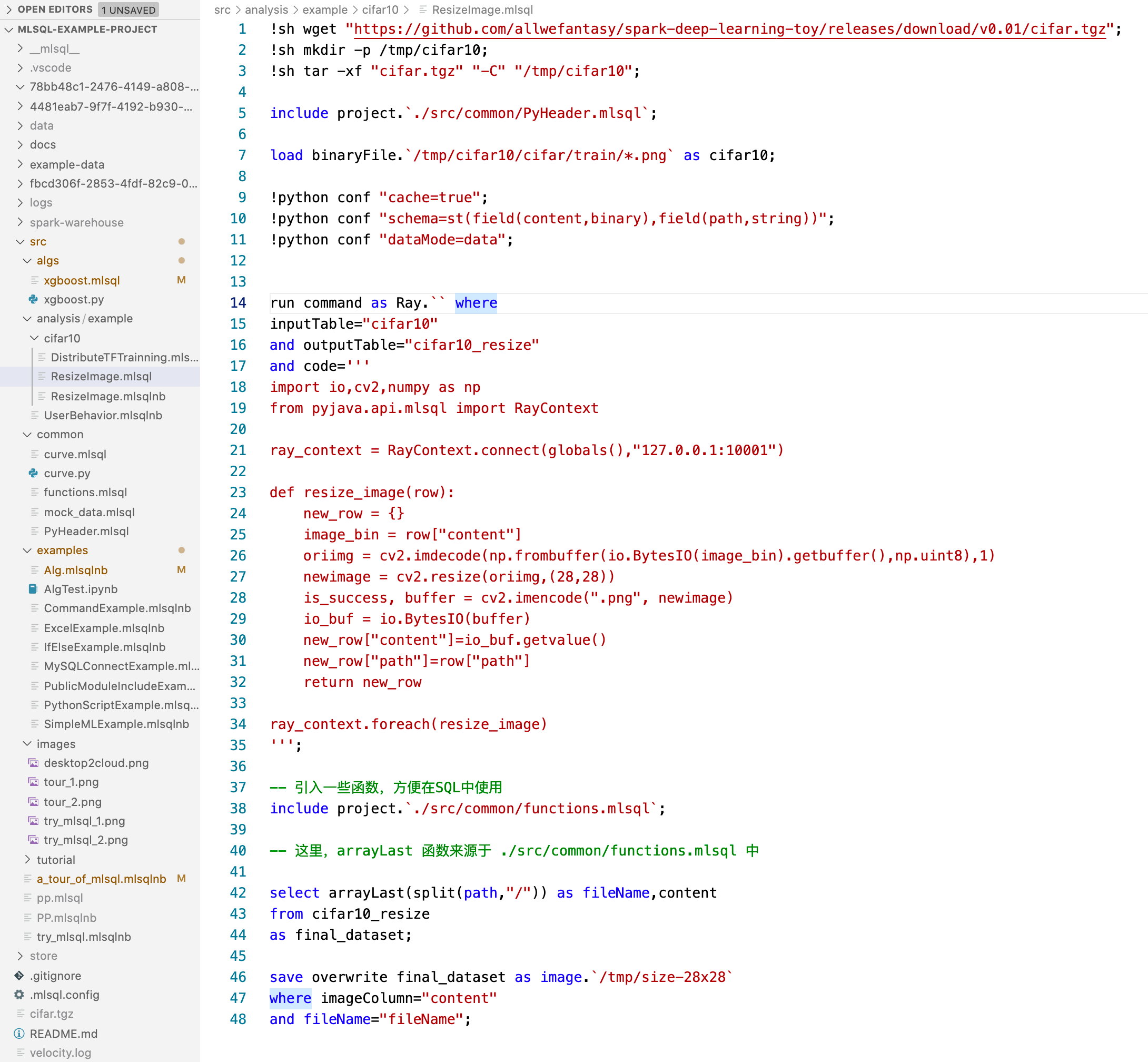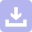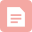# 可编程的SQL是什么样的？

## 背景

1. 保留SQL的所有原有优势，简洁易懂，上手就可以干活。
2. 允许用户进阶,提供更多可编程能力，但是以一种SQL Style的方式提供。

## 保留原有SQL精髓

load hive.raw.stripe_discounts as discounts;
load hive.raw.stripe_invoice_items as invoice_items;
select
invoice_items.*,
case
when discounts.discount_type = 'percent'
then amount * (1.0 - discounts.discount_value::float / 100)
else amount - discounts.discount_value
end as discounted_amount

from invoice_items

left outer join discounts
on invoice_items.customer_id = discounts.customer_id
and invoice_items.invoice_date > discounts.discount_start
and (invoice_items.invoice_date < discounts.discount_end
or discounts.discount_end is null) as joined;

select

id,
invoice_id,
customer_id,
coalesce(discounted_amount, amount) as discounted_amount,
currency,
description,
created_at,
deleted_at

from joined as final;

select * from final as output;


## 支持更多数据源

load excel../example-data/excel/hello_world.xlsx
as hello_world;

select hello from hello_world as output;


save overwrite hello_word as hive.tmp.excel_table;


## 变量

-- It takes effect since the declaration in the same cell.
set world="world";

select "hello ${world}" as title as output;  在可编程SQL中，变量支持多种类型，诸如sql,shell,conf,defaultParam等等去满足各种需求和场景。下面是一个典型的例子： set date=select date_sub(CAST(current_timestamp() as DATE), 1) as dt where type="sql"; select "${date}" as dt as output;


## 调用外部模块的代码

include lib.github.com/allwefantasy/lib-core
where
-- libMirror="gitee.com" and  -- 配置代理
-- commit="" and              -- 配置commit点
alias="libCore";


include local.libCore.udf.hello;
select hello() as name as output;


## 宏函数

set loadExcel = '''
load excel.{0}
as {1}
''';



3. 使用功能 ! 将loadExcel变量转化为宏函数进行调用。参数传递类似命令行。

set loadExcel = '''
load excel.${path} where header="true" as${tableName}
''';

!loadExcel _ -path ./example-data/excel/hello_world.xlsx -tableName helloTable;


## 原生SQL函数的动态扩展

register ScriptUDF. as arrayLast
where lang="scala"
and code='''def apply(a:Seq[String])={
a.last
}'''
and udfType="udf";

select arrayLast(array("a","b")) as lastChar as output;


## 分支语法

SQL最大的欠缺就是没有分支语句，这导致了一个啥问题呢？他需要寄生在其他语言之中，利用其他语言的分支语句。现在，我们原生的给SQL 加上了这个能力。 看如下代码：

set a = "wow,jack";

!if ''' split(:a,",") == "jack" ''';
select 1 as a as b;
!else;
select 2 as a as b;
!fi;

select * from b as output;


select 1 as a as mockTable;
set b_count=select count(*) from mockTable  where type="sql" and mode="runtime";

!if ''':b_count > 11 ''';

select 1 as a from b as final_table;
!else;
select 2 as a from b as final_table;
!fi;

select * from final_table as output;


## 机器学习（内置算法）

SQL表达机器学习其实是比较困难的。但是别忘了我们是可编程的SQL呀。我们来看看示例，第一步我们准备一些数据：

include project../src/common/mock_data.mlsql;
-- create mock/validate/test dataset.
select vec_dense(features) as features, label as label from mock_data as mock_data;
select * from mock_data as mock_data_validate;
select * from mock_data as mock_data_test;


train mock_data as RandomForest./tmp/models/randomforest where

keepVersion="true"

and evaluateTable="mock_data_validate"

and fitParam.0.labelCol="label"
and fitParam.0.featuresCol="features"
and fitParam.0.maxDepth="2"
;


predict mock_data_test as RandomForest./tmp/models/randomforest  as predicted_table;


register RandomForest./tmp/models/randomforest as model_predict;
select vec_array(model_predict(features)) as predicted_value from mock_data as output;


## Python脚本支持

select 1 as a as mockTable;

!python conf "schema=st(field(a,long))";

run command as Ray. where
inputTable="mockTable"
and outputTable="newMockTable"
and code='''
from pyjava.api.mlsql import RayContext

ray_context = RayContext.connect(globals(),None)

newrows = []
for row in ray_context.collect():
row["a"] = 2
newrows.append(row)

context.build_result(newrows)
''';

select * from newMockTable as output;


## 插件

!plugin app add - "mlsql-mllib-3.0";


run command as SampleDatasetExt.
where columns="id,features,label"
and size="100000"
and featuresSize="100"
and labelSize="2"
as mockData;

select * from mockData as output;


## 更多编程小trick

select SUM( case when id is null or id='' then 1 else 0 end ) as id,
SUM( case when diagnosis is null or diagnosis='' then 1 else 0 end ) as diagnosis,
SUM( case when radius_mean is null or radius_mean='' then 1 else 0 end ) as radius_mean,
SUM( case when texture_mean is null or texture_mean='' then 1 else 0 end ) as texture_mean,
SUM( case when perimeter_mean is null or perimeter_mean='' then 1 else 0 end ) as perimeter_mean,
SUM( case when area_mean is null or area_mean='' then 1 else 0 end ) as area_mean,
SUM( case when smoothness_mean is null or smoothness_mean='' then 1 else 0 end ) as smoothness_mean,
SUM( case when compactness_mean is null or compactness_mean='' then 1 else 0 end ) as compactness_mean,
SUM( case when concavity_mean is null or concavity_mean='' then 1 else 0 end ) as concavity_mean,
SUM( case when concave points_mean is null or concave points_mean='' then 1 else 0 end ) as concave_points_mean,
SUM( case when symmetry_mean is null or symmetry_mean='' then 1 else 0 end ) as symmetry_mean,
SUM( case when fractal_dimension_mean is null or fractal_dimension_mean='' then 1 else 0 end ) as fractal_dimension_mean,
SUM( case when radius_se is null or radius_se='' then 1 else 0 end ) as radius_se,
SUM( case when texture_se is null or texture_se='' then 1 else 0 end ) as texture_se,
SUM( case when perimeter_se is null or perimeter_se='' then 1 else 0 end ) as perimeter_se,
SUM( case when area_se is null or area_se='' then 1 else 0 end ) as area_se,
SUM( case when smoothness_se is null or smoothness_se='' then 1 else 0 end ) as smoothness_se,
SUM( case when compactness_se is null or compactness_se='' then 1 else 0 end ) as compactness_se,
SUM( case when concavity_se is null or concavity_se='' then 1 else 0 end ) as concavity_se,
SUM( case when concave points_se is null or concave points_se='' then 1 else 0 end ) as concave_points_se,
SUM( case when symmetry_se is null or symmetry_se='' then 1 else 0 end ) as symmetry_se,
SUM( case when fractal_dimension_se is null or fractal_dimension_se='' then 1 else 0 end ) as fractal_dimension_se,
SUM( case when radius_worst is null or radius_worst='' then 1 else 0 end ) as radius_worst,
SUM( case when texture_worst is null or texture_worst='' then 1 else 0 end ) as texture_worst,
SUM( case when perimeter_worst is null or perimeter_worst='' then 1 else 0 end ) as perimeter_worst,
SUM( case when area_worst is null or area_worst='' then 1 else 0 end ) as area_worst,
SUM( case when smoothness_worst is null or smoothness_worst='' then 1 else 0 end ) as smoothness_worst,
SUM( case when compactness_worst is null or compactness_worst='' then 1 else 0 end ) as compactness_worst,
SUM( case when concavity_worst is null or concavity_worst='' then 1 else 0 end ) as concavity_worst,
SUM( case when concave points_worst is null or concave points_worst='' then 1 else 0 end ) as concave_points_worst,
SUM( case when symmetry_worst is null or symmetry_worst='' then 1 else 0 end ) as symmetry_worst,
SUM( case when fractal_dimension_worst is null or fractal_dimension_worst='' then 1 else 0 end ) as fractal_dimension_worst,
SUM( case when _c32 is null or _c32='' then 1 else 0 end ) as _c32
from data as data_id;


select
#set($colums=["id","diagnosis"，"fractal_dimension_worst"]) #foreach($column in $colums ) SUM( case when $column is null or $column='' then 1 else 0 end ) as$column,
#end
1 as a from newTable as output;


set sum_tpl = '''
SUM( case when {0} is null or {0}='' then 1 else 0 end ) as {0}
''';

select ${template.get("sum_tpl","diagnosis")},${template.get("sum_tpl","radius_mean")},
\${template.get("sum_tpl","texture_mean")},
from data as output;


## 真的最后了

MLSQL目前支持Web版，桌面版，包括Script,Notebook等多种交互模式。参考 MLSQL 2.1.0版本的技术白皮书

1. 下载图片tar包，并且解压
2. 设置python环境
3. 加载图片目录为表
4. 使用python进行分布式图片处理
5. 对文件名进行处理
6. 将表以二进制图片包保存到对象存储目录中下载

06-29博客
SQL Server基础教程(二)数据库对象：表、视图、同义词、可编程、外部资源、Service Broker、存储、安全 分别表示什么意思
12-1068博客

05-0865博客

05-11132博客
SQL函数和可编程
04-19490博客

11-20344博客
SQL server数据库可编程要点
11-17401博客
SQL Server 2008综合数据可编程
01-16124博客

05-1665博客

05-182148博客
SQL Server 2012可编程新特_1_Sequence
03-261555博客
Sqlserver(可编程对象)
10-21166博客
Oracle基础(十二)可编程SQL
09-05195博客
Android初学之十六：使用SQL操作数据库
05-2638博客
PowerBI数据市场预览
05-2647博客
MySQL----数据库概述
05-2853博客
220416-oracle-基础常用关键字

05-285博客
TiDB数据库读取慢排查分析
05-2712

### “相关推荐”对你有帮助么？

•非常没帮助
•没帮助
•一般
•有帮助
•非常有帮助¥2 ¥4 ¥6 ¥10 ¥20余额支付 (余额：-- )扫码支付获取中扫码支付点击重新获取扫码支付1.余额是钱包充值的虚拟货币，按照1:1的比例进行支付金额的抵扣。
2.余额无法直接购买下载，可以购买VIP、C币套餐、付费专栏及课程。余额充值